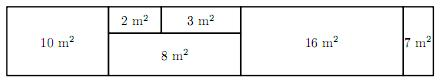favorite We need a little bit of your help to keep things running, click on this banner to learn more
Competitions

# Flat

You are one of the developers of software for a real estate agency. One of the functions you are to implement is calculating different kinds of statistics for flats the agency is selling. Each flat consists of different types of rooms: bedroom, bathroom, kitchen, balcony and others.

The cost of the flat is equal to the product of reduced total area and the cost of one square meter. Reduced total area is the total area of all rooms except for balconies plus one half of balconies total area.

You will be given some information about the area of each room in the flat and the cost of one square meter. You are to calculate the following values for the flat:

• the total area of all rooms;
• the total area of all bedrooms;
• the cost of the flat.

#### Input

The first line contains two integer numbers n (1n10) and c (1c100000) - number of rooms in the flat and the cost of one square meter, respectively.

Each of the following n lines contains an integer number `ai` (1`ai`100) and a word `ti` - the area of i-th room and its type, respectively. Word `ti` is one of the following: "bedroom", "bathroom", "kitchen", "balcony", "other".

#### Output

The first line should contain one integer number - the total area of all rooms of the flat. The second line should contain one integer number - the total area of bedrooms of the flat. The third line should contain one real number - the cost of the flat with precision not worse than `10-6`.

The figure shows the flat from the first example.Time limit 1 second
Memory limit 128 MiB
Input example #1
```6 75000
8 other
3 bathroom
2 bathroom
10 kitchen
16 bedroom
7 balcony
```
Output example #1
```46
16
3187500.0
```
Input example #2
```2 75123
10 kitchen
15 balcony
```
Output example #2
```25
0
1314652.5
```
Source 2011 NEERC, Northern Subregion, October 29, Problem F